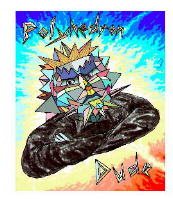Acronym siddo Name snub disoctahedron,octahedral compound of 2 ike,vertex figure of sody `© ©©` Circumradius sqrt[(5+sqrt(5))/8] = 0.951057 Inradius sqrt[(7+3 sqrt(5))/24] = 0.755761 Coordinates (τ/2, 1/2, 0)   & all permutations, all changes of sign where τ = (1+sqrt(5))/2 Vertex figure  General of army x3f4o Colonel of regiment (is itself locally convex) Dihedral angles (at margins) between {3} and {3}:   arccos(-sqrt(5)/3) = 138.189685° ExternallinksFaces of an octahedral subset of triangles pairwise fall into coincident face planes. So either those can be considered separately (type A); or they are considered as (rotated) 2-triangle-compounds (type B).

The right pic above displays a coloring induced as outer symmetry when being used as vertex figure of sody (for further details see there).

Incidence matrix according to Dynkin symbol

```β3β4o   (Type A)

both( . . . )    | 24 |  1  4 |  2  3 || 1
-----------------+----+-------+-------++--
both( . s4o )    |  2 | 12  * |  0  2 || 1
sefa( β3β . )  & |  2 |  * 48 |  1  1 || 1
-----------------+----+-------+-------++--
both( s3s . )  & |  3 |  0  3 | 16  * || 1
sefa( β3β4o )    |  3 |  1  2 |  * 24 || 1
-----------------+----+-------+-------++--
both( s3s4o )    ♦ 12 |  6 24 |  8 12 || 2

starting figure: x3x4o
```

```
β3β4o   (Type B)

both( . . . )    | 24 |  1  4 | 2  3 || 1
-----------------+----+-------+------++--
both( . s4o )    |  2 | 12  * | 0  2 || 1
sefa( β3β . )  & |  2 |  * 48 | 1  1 || 1
-----------------+----+-------+------++--
β3β .    & |  6 |  0  6 | 8  * || 2
sefa( β3β4o )    |  3 |  1  2 | * 24 || 1
-----------------+----+-------+------++--
both( s3s4o )    ♦ 12 |  6 24 | 8 12 || 2

starting figure: x3x4o
```

```β3β3β   (Type A)

both( . . . )    | 24 |  1  4 |  2  3 || 1
-----------------+----+-------+-------++--
both( s 2 s )    |  2 | 12  * |  0  2 || 1
sefa( β3β . )  & |  2 |  * 48 |  1  1 || 1
-----------------+----+-------+-------++--
both( s3s . )  & |  3 |  0  3 | 16  * || 1
sefa( β3β3β )    |  3 |  1  2 |  * 24 || 1
-----------------+----+-------+-------++--
both( s3s3s )    ♦ 12 |  6 24 |  8 12 || 2

starting figure: x3x3x
```

```
β3β3β   (Type B)

both( . . . )    | 24 |  1  4 | 2  3 || 1
-----------------+----+-------+------++--
both( s 2 s )    |  2 | 12  * | 0  2 || 1
sefa( β3β . )  & |  2 |  * 48 | 1  1 || 1
-----------------+----+-------+------++--
β3β .    & |  6 |  0  6 | 8  * || 2
sefa( β3β3β )    |  3 |  1  2 | * 24 || 1
-----------------+----+-------+------++--
both( s3s3s )    ♦ 12 |  6 24 | 8 12 || 2

starting figure: x3x3x
```